﻿ Maxime Euzière

## codegolfctober 2018

### October 2018

Like last year, the codegolf team and I decided to show one codegolf thing a day for the whole month of October!

Most were new, but we also showed our favourite demos from last JS1k, JS13k and Dwitter.

Thanks to Aemkei, ETHprod, Justecorruptio, BalintCsala, Zozuar, Xen_the, Zlprp, Subzey, P01, Keithclarkcouk, Xaotic, Veubeke, Tomxor, JS1K and Dwitter for contributing to this month's awesomeness!

## Day 1: Nano Unicode Slideshow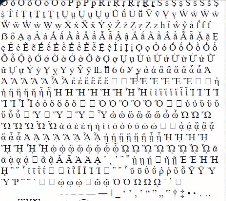``````i=!setInterval(`document.body.innerHTML="&#"+i++`,99) // 53b
i=!setInterval`document.write(" &#"+i++)` // 41b, Webkit only``````

We reduced the "nano" demo of MiniUnicode from 54b to 53b / 41b.

The 53b version just changes how i is initialized ("i=!setInterval" instead of "i=0;setInterval")

The 41b version abuses the fact that Webkit allows to call setInterval without second parameter, and to call document.write repeatedly, which makes non-Webkit browsers freeze.

## Day 2: ASCII Game of Life``<body onload=b=!setInterval`for(a=b,b=[h=i=985];i--;_.innerText=h+="▁▉-"[i%42?b[i]=a?d<9&7-a[i]<d:i<69:2])for(d=j=5;e=(2<j--)*39+j;)d+=a[i+e]+a[i-e]` id=_>``

We reduced an old Game of Life drawn in ASCII down to 165 then 159b.

The bytes saved come from a new way to compute the state of a cell based on the number of alive neighbours.

## Days 3 & 4: mini 2D vector library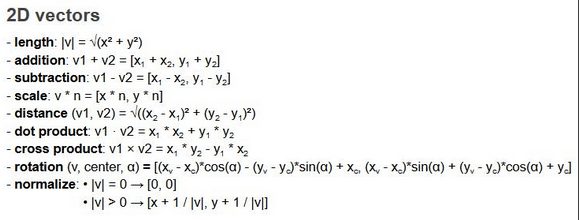``````// API
//- vec2(x,y)
//- length(v)
//- sub(v,w)
//- mul(v,n)
//- dist(v,w)
//- dotproduct(v,w)
//- crossproduct(v,w)
//- rotate(v,origin,theta)
//- normalize(v)

// Not regpacked version (264b)
V=(x,y)=>({x,y})
l=v=>d(v,v)**.5
a=(v,w)=>V(v.x+w.x,v.y+w.y)
s=(v,w)=>a(v,m(w,-1))
m=(v,n)=>V(v.x*n,v.y*n)
t=(v,w)=>l(s(v,w))
d=(v,w)=>v.x*w.x+v.y*w.y
c=(v,w)=>v.x*w.y-v.y*w.x
r=(v,o,t)=>a(o,V(c(f=s(v,o),g=V(Math.sin(t),Math.cos(t))),d(f,g)))
n=v=>m(v,1/(l(v)||1))``````

We had fun golfing this lib of 10 functions in 264b, by using functions inside other functions as much as possible to save bytes.

For example, the distance between two vectors is "length(sub(v,w))" instead of Math.sqrt(v.x-w.y, v.y-w.y).

And sub is also reusing add and mul instead of redoing the whole operation.

And length(v) returns the square root of dot(v, v), which corresponds to v.x² + v.y². Etc...

I'm very impressed by the solution they came up with for rotate, but I gave up trying to understand it ^^

``````// Regpackable version (236b regpacked)
v=(x,y,t)=>({x,y});
l=(x,y,t)=>d(x,x)**.5;
a=(x,y,t)=>v(x.x+y.x,x.y+y.y);
s=(x,y,t)=>a(x,m(y,-1));
m=(x,y,t)=>v(x.x*y,x.y*y);
t=(x,y,t)=>l(a(x,m(y,-1)));
d=(x,y,t)=>x.x*y.x+x.y*y.y;
c=(x,y,t)=>x.x*y.y-x.y*y.x;
r=(x,y,t)=>a(y,v(c(a(x,m(y,-1)),v(Math.sin(t),Math.cos(t))),d(a(x,m(y,-1)),v(Math.sin(t),Math.cos(t)))));
n=(x,y,t)=>m(x,1/(l(x)||1));``````

The next day, we optimized it to be as compressible by Regpack as possible, by repeating signatures, semicolons, and expanding some factorizations to maximize repetitions. The result fits in 236b.

## Day 5: Mini Music Player

``with(new AudioContext)with(G=createGain())for(i in D=[...Array(26).keys()])with(createOscillator())if(D[i])connect(G),G.connect(destination),start(i*.2),frequency[v="setValueAtTime"](440*1.06**(13-D[i]),i*.2),gain[v](1,i*.2),gain.setTargetAtTime(.001,i*.2+.18,.005),stop(i*.2+.19)``

A tiny music player, including the output of MiniMusic (golfed during js13kgames 2017 and 2018 and regolfed here in 262 bytes) combined with the smallest code that generates an array from 1 to N (gorfed during the day 10 of golfctober 2017, 20 bytes).

The result is a 280b script playing 2 octaves in decrescendo, while avoiding to make a "click" at the end of each note, that Oscillators do by default when we stop them abruptly.

(warning, the volume is loud by default)

## Day 6: Strange Crystals v0.140b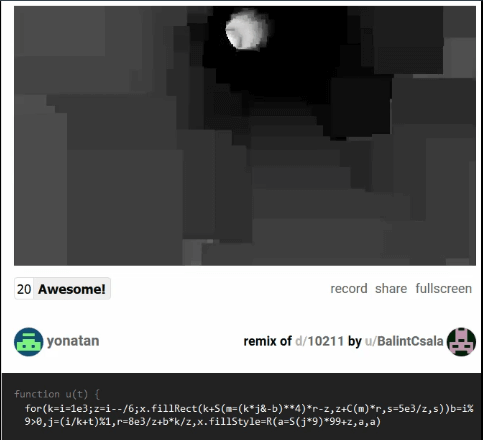``for(k=i=1e3;z=i--/6;x.fillRect(k+S(m=(k*j&-b)**4)*r-z,z+C(m)*r,s=5e3/z,s))b=i%9>0,j=(i/k+t)%1,r=8e3/z+b*k/z,x.fillStyle=R(a=S(j*9)*99+z,a,a)``

@BalintCsala and @zozuar golfed a 140b demake of Strange Crystals II (winning js1k 2013 entry by @ehouais).

@zozuar wrote an amazing writeup about it here.

## Days 7 & 8 & 11 & 16 & 30: Mini Serpinski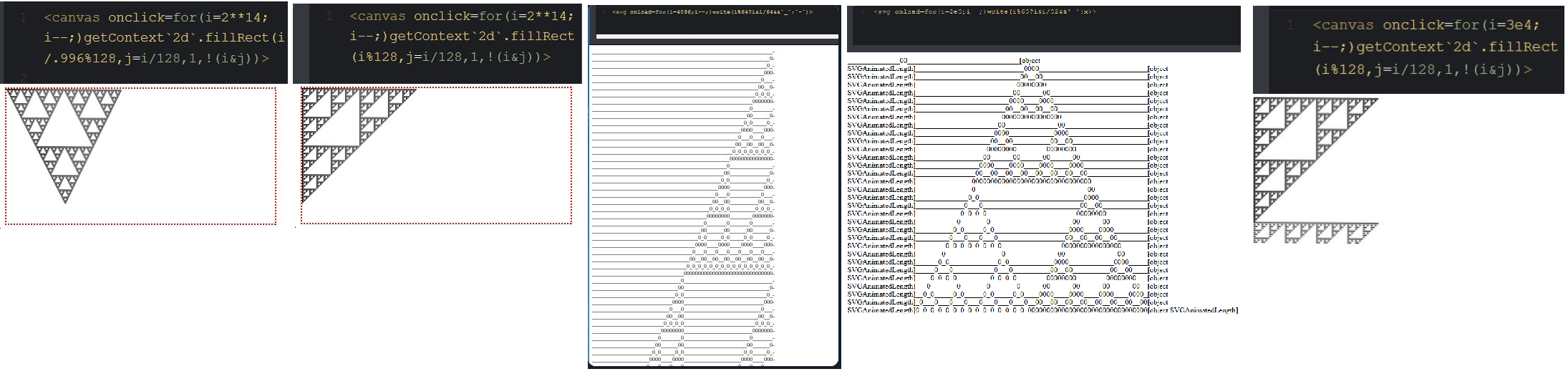During this month, most of the time on Slask was used to golf mini Serpinski triangles on canvas and in ASCII, straight or lopsided, centered or right-aligned. Here are the different achievements of the team:

``<canvas onclick=for(i=2**14;i--;)getContext`2d`.fillRect(i/.996%128,j=i/128,1,!(i&j))>``
``<canvas onclick=for(i=2**14;i--;)getContext`2d`.fillRect(i%128,j=i/128,1,!(i&j))>``
``<svg onload=for(i=4096;i--;)write(i%64?i&i/64&&`_`:`-`)>``
``<svg onload=for(i=2e3;i--;)write(i%63?i&i/32&&'_':x)>``
``<canvas onclick=for(i=3e4;i--;)getContext`2d`.fillRect(i%128,j=i/128,1,!(i&j))>``

## Days 9 & 10 & 12 & 13: MiniCalculator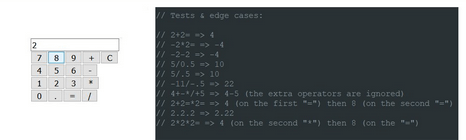A wild codegolf project appeared on day 9, based on an idea prototyped by @ETHprod: a mini calculator!

During the following week, we golfed it in two different versions: full-featured and minimalist.

It was pretty fun to golf the generation of the UI (input, buttons), while implementing each button's features, while precisely matching the behavior of a real calculator in every possible edge case. Boy, those things are weird, but our code is even weirder!

``````<!-- Full-featured calc -->
<body onload='for(s of"C/=.0 *321 -654 +987 ")i.outerHTML+=s<"!"?"<p>":`<button onclick=F("\${s}")>`+s;F=b=>i.value=z=b>"B"?(c=d=o="",a=r=p=0):1+b-0?c+=b-.1?b:d?"":d=b:(q=b<"=",p?r=c:a=c||a,r=q?p&&r:r||a,a=o&&r?z=eval(a+o+s+r):a,o=q?b:o,p=q,d=c="",q?z:a);F`C`'><input id=i>``````
``<input id=i><script>o='';for(s of"C/=.0!*321!-654 +987!")i.outerHTML+=s<"#"?"<p>":`<button onclick="o=i.value=\${s=="="?`eval(o)`:s>"B"?`''`:`o+'\${s}'`}">`+s</script> ``

## Days 14, 15: MiniSweeper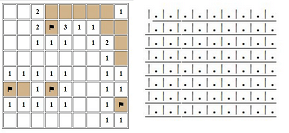My oldest codegolfed game, made in 497b with Subzey back in 2013, lost 11 bytes after I used ES6 and removed old browsers hacks.

``<table border id=t><script>b=[v=[]];f=[l=g=0];n=[];r=d=>{for(M=!l;M;l=g)for(a=M=H="";s>++a;)for(j=s,H+="<tr>";~--j;H+=`<th width=25 height=30 onclick=b[i=\${I}]?g--:v[i]=1;r() oncontextmenu=for(f[\${I}]^=g=1,a=0;a<s*s;)g&=b[a]^!f[a++];return!!r() \${(v[I]|g||"bgcolor=tan")}>`+(f[I]?"⚑":b[I]&g?"💣":v[I]|g&&n[I]||""))for(I=a*s+j,T=d?b[I]=.1>Math.random():0,x=2;~x--;)for(y=2;~y--;~B&&B<s?(n[C]=~~n[C]+T)||v[I]|b[I]|!v[C]?0:v[I]=M=1:0)B=j+y,C=(a+x)*s+B;t.innerHTML=H};r(s=9)</script>``

Then Aemkei joined the party and brought it down to 349b while converting it to ASCII art, which means 148 bytes saved!

``````<pre id=t><script>b=[v=[]];l=g=0;n=[];r=d=>{for(M=!l;M;l=g)for(a=M=H="";s>++a;)for(j=s,H+=`
`;~--j;t.innerHTML=H+=`|<u onclick=b[i=\${I}]?g--:v[i]=1;r()>`+(b[I]&g?"#":v[I]|g&&n[I]||(v[I]|g?" ":'.')))for(I=a*s+j,T=d?b[I]=.1>Math.random():0,x=2;~x--;)for(y=2;~y--;~B&&B<s?(n[C]=~~n[C]+T)||v[I]|b[I]|!v[C]?0:v[I]=M=1:0)B=j+y,C=(a+x)*s+B};r(s=9)</script>``````

## Day 17: Mini Keyboard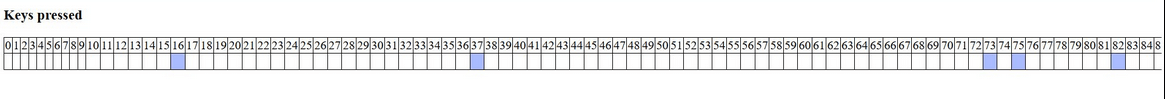``k={};onkeydown=onkeyup=e=>k[e.which]=e.type``

## Day 18: Mini Simon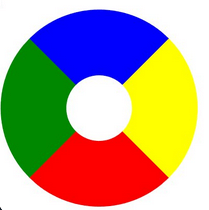I regolfed our old Simon game and saved 53 bytes by removing old browser hacks and applying the new sound generation techniques from day 5, but even more simplified, because only four different beeps were required.

The frequencies of the buttons k=0 to k=3 are computed with "frequency.value=99+k*99". The first 99 makes the sound "0" audible, and the second 99 makes the sounds different enough from each other.

``<body onload="a=(k,q)=>{z=['00F','FF0','F00','080'];if(~k){with(new AudioContext)with(createOscillator())start(0,connect(destination),frequency.value=99+k*99)+stop(.2,s(a,250,-1));z[(3+k)%4]=111};p.style.borderColor='#'+z.join`#`;if(q)k^f[j]?p.outerHTML=':(':++j^g||h()};(h=e=>{for(i=g=f.push(new Date%4);j=i;s(a,500*i--,f[i]))s=setTimeout})(f=[])"onkeyup=a(event.keyCode-37,1) style="width:9em;height:9em;border-radius:50%;border:9em solid"id=p>``

## Day 19: Print all the chars that the program doesn't contain

Our first attempt back in 2013 took 88 bytes, this tiime it's down to 74b, due to a completely different approach involving ES6 and very hacky things.

``eval(v="for(i=32;i<127;)v.includes(e=String.fromCharCode(i++))||alert(e)")``

## Day 20: Char generation

We explored many ways to generate a char from an integer, to improve day 19's challenge. As far as we know, there are 4 solutions below 30 bytes, but none of them beats String.fromCharCode(i)...

## Day 21: Space dweets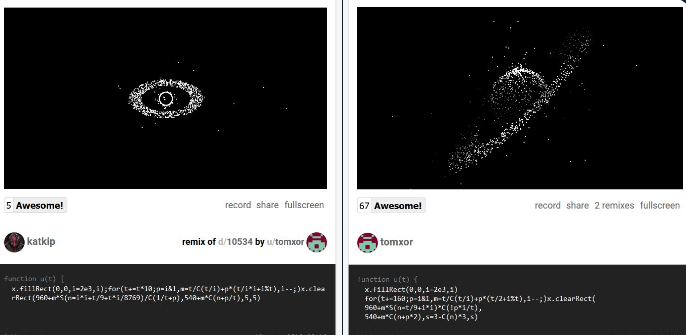Promoting some great dweets of Tomxor:
Black Hole: DEMO
Saturn: DEMO

## Day 22: Mini Split ScreenI had done this little split screen browser app last year, using 2, 3, 4 or 6 iframes to avoid having many browser windows in parallel on the same screen, and preventing one of the iframes to change the URL of the parent window.

Now it's golfed in 225b, and with a lot of weird CSS to make it look nice on any screen size!

``<body onbeforeunload=return!1><input oninput=c.src=value><input oninput=d.src=value><iframe id=c></iframe><iframe id=d></iframe><style>*{margin:0;padding:0;box-sizing:border-box}body *{width:50%}#c,#d{height:calc(100vh - 20px``

## Day 23: Mini Sound Player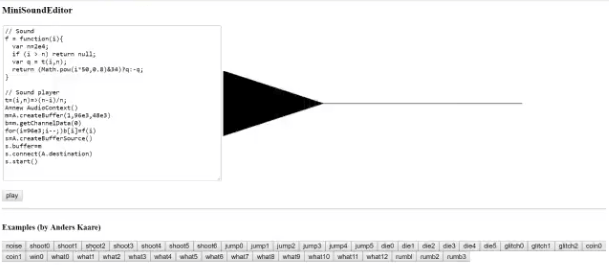I took the sound player (and sound collection) that Anders Kaare made for our js13k16 and 17 entries, and gathered them in an app that's able to edit, export, play and even draw each sound with a 2-second limit. I also golfed the player in 176b and the result is below. It needs a custom function f(i) that gives the value of each sample.

``````t=(i,n)=>(n-i)/n;
A=new AudioContext()
m=A.createBuffer(1,96e3,48e3)
b=m.getChannelData(0)
for(i=96e3;i--;)b[i]=f(i)
s=A.createBufferSource()
s.buffer=m
s.connect(A.destination)
s.start()``````

## Day 24: Mega Million Picker

The Mega Million lottery happened that month in the USA and people on Twitter tried to make a Mega Million number picker, with specific rules (Picks 5 unique ordered numbers between 1 and 70, then 1 number between 1 and 25).

We joined them and made this version in 88b and a lot of rarely used before ES6 features.

``[z,...a]=Array(71).keys(),[...(f=_=>a?f(a.splice(Math.random(z++)*99,1)):a)(),z%25+1]``

## Day 25: Mini LOSSSTA little throwback to our 1kb LOSSST demake (a.k.a. SN1KE) made during JS1K 2017.

## Days 26 and 27: Mini Fourier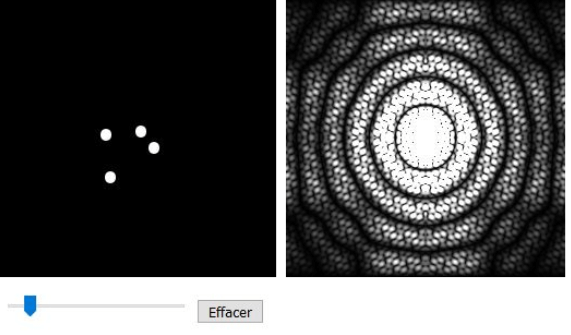Another js1k 2017 throwback with this instant 2D FFT tracer.

The next day, we isolated and golfed the 1D FFT function from this js1k entry, in 312b.

``F=(e,f)=>{M=Math;n=e.length;for(i=0;i<n;i++){for(j=0,h=i,k=n;k>>=1;h>>=1)j=j<<1|h&1;j>i&&([e[j],e[i],f[j],f[i]]=[e[i],e[j],f[i],f[j]])}for(h=1;2*h<=n;h*=2)for(i=0;i<n;i+=2*h)for(j=i;j<i+h;j++)l=M.cos(k=M.PI*(j-i)/h),m=M.sin(k),o=e[j+h]*l+f[j+h]*m,p=-e[j+h]*m+f[j+h]*l,e[j+h]=e[j]-o,f[j+h]=f[j]-p,e[j]+=o,f[j]+=p}``

## Days 28 & 29: Epic Cycles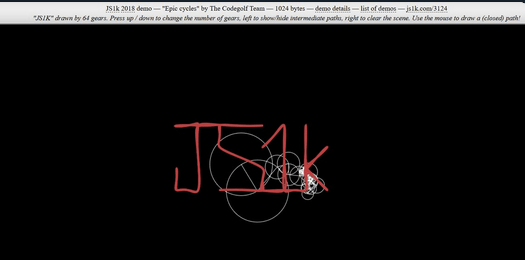Final throwback to JS1K 2017 with our 1kb epicycloid tracer

The next day; we isolated the 1D DFT function from this entry (simpler than a FFT, but slower), and golfed it in 161b

``D=n=>{for(i in _=[],M=Math,n)for(k in _[i]=[0,0],n)b=M.cos(a=-2*k*M.PI*i/n.length),c=M.sin(a),_[i]+=n[k]*b-n[k]*c,_[i]+=n[k]*b+n[k]*c;return _}``

## Day 31: Mini Peach CastleThe first sneak peek of my WIP entry for JS1K 2019: Mario 64's Peach Castle, as complete as possible, in 1kb, and rendered with HTML and CSS3D. More details soon!